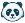# [New Quizzes] Partial credit for formula questions

Problem statement:

Formula questions currently are either right or wrong. Partial credit should be able to be awarded if students have gone some way to answering the question. For example:

A kettle rated `POWER` W takes `TIME` minutes to boil water. How much energy does the kettle transfer in Joules?

Partial credit answer = POWER * TIME //Time not converted, but correct equation used

Proposed solution:

Allow for extra answers by having more "formula definition" fields. Different marks awarded for each, and different feedback for each. Another solution involves using pre-existing formula definition field. You can define multiple formulas to help compute an answer already. So, these mid-way formulas could be worth partial credit.

User role(s):